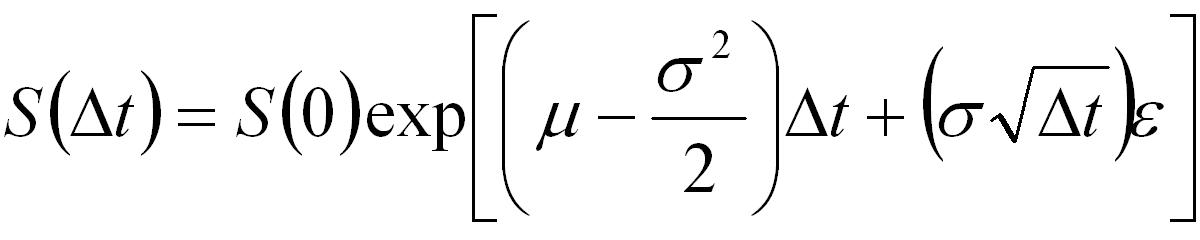## Using Antithetic Variates in MATLAB

This tutorial presents MATLAB code that generates simulated asset paths using the antithetic variates form for variance reduction and then uses them to price an Asian option. A general discussion of Monte-Carlo simulation is presented in the Monte-Carlo Methods tutorial, and the mathematical background to antithetic variates is given in the Variance Reduction tutorial.

Note that the primary purpose of the code presented here is to show how to efficiently generate the simulation paths and price the option. The code contains no error checking and as such it is not suitable for inclusion into a larger application without appropriate modifications.

### MATLAB Function: AssetPathsAntithetic

The following is code for generating a user specified number of simulated asset paths using antithetic variates and assuming the asset follows the standard log-normal/geometric Brownian motion model,Equation 1: Stock Price Evolution Equation

```function S = AssetPathsAntithetic(S0,mu,sig,dt,steps,nsims)
% Function to generate sample paths using antithetic variates
% and for assets assuming geometric Brownian motion.
% When epsilon is a vector of random numbers used to generate
% one simulated path then -epsilon is used to generate another
% path.
%
% S = AssetPathsAntithetic(S0,mu,sig,dt,steps,nsims)
%
% Inputs: S0 - stock price
%       : mu - expected return
%       : sig - volatility
%       : dt - size of time steps
%       : steps - number of time steps to calculate
%       : nsims - (even) number of simulation paths to generate
%
% Output: S - a matrix where each column represents a simulated
%             asset price path.
%
% Notes: This code focuses on details of the implementation of the
%        Monte-Carlo algorithm.
%        It does not contain any programatic essentials such as error
%        checking.
%        It does not allow for optional/default input arguments.
%        It is not optimized for memory efficiency or speed.

% Author: Phil Goddard (phil@goddardconsulting.ca)
% Date: Q2, 2006

% calculate the drift
nu = mu - sig*sig/2;

% generate random samples
epsilon = randn(steps,nsims/2);

% Generate potential paths
S = S0*[ones(1,nsims); ...
cumprod(exp(nu*dt+sig*sqrt(dt)*[epsilon -epsilon]),1)];
```

### Example Usage

The following MATLAB code gives an example of how to use the function AssetPathsAntithetic, to price an Asian option with payoff given byEquation 2: Payoff for an Asian Put and Call Option

where A is the average price of the underlying asset over the life of the option and X is the strike.

```% Script to price an Asian option using a monte-carlo approach.

S0 =50;       % Price of underlying today
X = 50;       % Strike at expiry
mu = 0.04;    % expected return
sig = 0.1;    % expected vol.
r = 0.03;     % Risk free rate
dt = 1/365;   % time steps
etime = 50;   % days to expiry
T = dt*etime; % years to expiry

nruns = 100000; % Number of simulated paths

% Generate potential future asset paths
S = AssetPathsAntithetic(S0,mu,sig,dt,etime,nruns);

% calculate the payoff for each path for a Put
PutPayoffT = max(X-mean(S),0);

% calculate the payoff for each path for a Call
CallPayoffT = max(mean(S)-X,0);

% discount back
putPrice = mean(PutPayoffT)*exp(-r*T)
callPrice = mean(CallPayoffT)*exp(-r*T)
```

The result of executing the above script is,

```putPrice =
0.3590
callPrice =
0.4958
```

An example of pricing the same option without using antithetic variates is available in the Pricing an Asian Option in MATLAB tutorial. Other MATLAB based Monte-Carlo tutorials are linked off the Software Tutorials page.